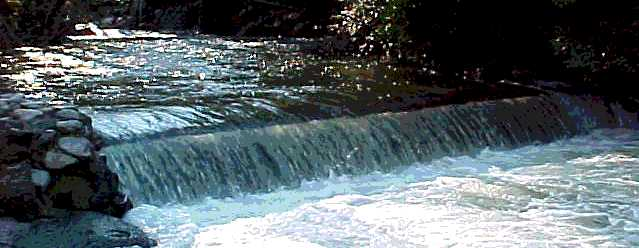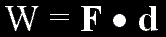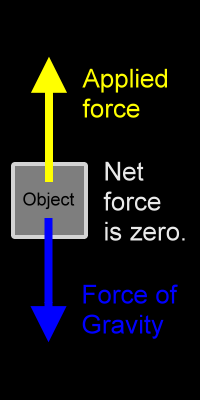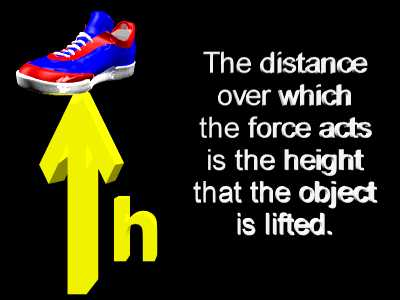﻿ Gravitational Potential Energy | Zona Land Education

# Gravitational Potential EnergyThe water above receives energy as it falls down the short waterfall. This energy was stored as potential energy in the gravitational field of the Earth and came out of storage as the water dropped. This energy which came out of the gravitational field ended up being expressed as the kinetic energy of the water. That is, the water gains kinetic energy as it drops. An ounce of water is going faster when it hits the bottom of the waterfall than it was when it went over the top of the waterfall.

First Definition

Energy that is stored in the gravitational field is called gravitational potential energy, or potential energy due to gravity.

Formula For Work

Let's see how energy gets stored in the gravitational field.

First of all, remember that work equals force times distance as stated in this formula:The above formula for work shows a dot product for the multiplication of the force vector times the displacement vector. If the force and displacement vectors are in the same direction, then this boils down to a simple multiplication of the size of the force times the size of the displacement. This will be the case for our discussion here.

Force Doing The Lifting

Now, let's consider what happens when an object is being lifted. We will consider lifting an object at constant velocity. That is, during the lift the object will not be accelerating. For the moment we will not discuss the very start of the lift, when the object must begin to move upward from a stationary position. Nor will we, for the moment at least, discuss the very end of the lift, when the object must slow to a stop after the upward motion is finished. Here, we are talking about the motion during the lift, and we will consider this motion to be directly upward and at constant speed.If the object is being lifted at constant velocity, then it is not accelerating, and the net force on it is zero. We know that from Newton's first law of motion.

We must pull up on an object when we lift it. So, the agent doing the lifting must provide an upward force on the object. This would be the yellow applied force in the animation at left.

Gravity, of course, will be pulling down. This pull down of gravity is called the weight of the object. It is the blue downward force in the animation at left.

If during the lift the net force on the object is zero, then the upward pull must be canceled by the downward pull. That is, the upward lifting force must be equal in size to the downward pull of gravity.

In summary, when you are lifting something at constant velocity, the upward pull that you provide is equal to the weight of the object.

When lifting something at a constant velocity....

The force that you lift with (applied force)

Equals

The weight of the object (gravitational force)

Distance For The Lift

Over what distance does this force act? Well, that would be the distance over which you lift the object. That distance we will call the height of the lift.Work Done LiftingNow, work is force times displacement. The force that you apply is equal to the weight of the object. The weight of the object is its mass times the acceleration due to gravity. And the displacement over which this work is done is the height of the lift.

So, the work done lifting an object is equal to its mass times the acceleration due to gravity times the height of the lift. This is summarized below:

 W= Fd Work equals the applied force times displacement. W= Fgd The applied force equals the weight of the object. (F = Fg) W= mgd The weight of the object equals its mass times the acceleration due to gravity. (Fg = mg) W= mgh The displacement over which the work is done equals the height of the lift. (d = h)

Work Becomes Stored Energy

This work which is done on the object as it is lifted does not end up as energy of motion, or kinetic energy, since, after the lift, the object is not moving; it is stationary. It is higher up, though. We say that the energy has been stored in the gravitational field. This stored energy is called potential energy. Since it is energy in a gravitational field, it is called gravitational potential energy or potential energy due to gravity.

The symbol for potential energy, in general, is U, and the symbol for gravitational potential energy is Ug.

 Ug Symbol for gravitational potential energy

Since the work done on the object when it is lifted becomes the gravitational potential energy, the formula for gravitational potential energy equals the massif the object times the acceleration due to gravity times the height that the object is lifted, as in:

Ug= mgh

Sample Calculation

What is the gravitational potential energy for a 4 kg object that is lifted 5 m?

 Ug = mgh Formula for gravitational potential energy. Ug = (4 kg)(9.8 m/s/s)(5 m) Plug in values for mass, acceleration due to gravity, and height. Ug = 196 J Gravitational potential energy equals 196 Joules.

Questions

Here are some questions to work with using the above formula.

Release Of Potential Energy

Once the work has been done lifting the object, and the energy has been stored in the gravitational field, how is this potential energy released? Very simply, the object can be dropped. As it falls its height above ground gets less and less, so, the potential energy stored in gravity gets less and less, also. The object, though, is speeding up as it falls. Energy is leaving the gravitational field and entering the falling object as kinetic energy. Kinetic energy is the energy of motion.

As the object falls it travels faster and faster, and thus, picks up more and more kinetic energy. This increase in kinetic energy during the fall is due to the drop in gravitational potential energy during the fall. The gravitational potential energy becomes the kinetic energy of the falling object.

Energy Of Position

Potential energy is often described as stored energy, and this is a fine description. However, a more sophisticated definition would be that potential energy is the energy of position, or energy related to, or as a function of, position.

Consider the two flower pots below. The one on the left is on the floor at ground level. The one on the right is several centimeters above ground level.Now, the one on the right is at a higher gravitational potential energy than the one on the left. Of course, it is at a higher position. So, the potential energy that each pot has is a function of its vertical position. In fact, for any one mass on any one planet, the vertical position determines the amount of gravitational potential energy. Other forms of potential energy are dependent upon position also. That is why potential energy is often called the energy of position.